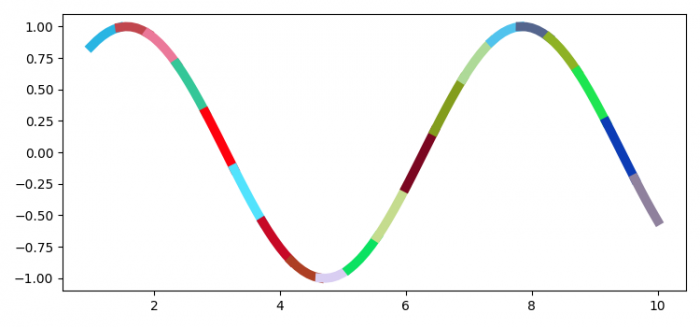# How to plot a single line in Matplotlib that continuously changes color?

To plot a single line that continuously changes color, we can take the following steps−

• Set the figure size and adjust the padding between and around the subplots.
• Create random x and y data points using numpy.
• Create a figure and a set of subplots.
• Iterate the index in the range of 1 to 100.
• Plot x and y data points with random color in a loop.
• To display the figure, use show() method.

## Example

import matplotlib.pyplot as plt
import numpy as np
import random

plt.rcParams["figure.figsize"] = [7.50, 3.50]
plt.rcParams["figure.autolayout"] = True

x = np.linspace(1, 10, 100)
y = np.sin(x)
fig, ax = plt.subplots()

for i in range(0, 100, 5):
r = random.random()
b = random.random()
g = random.random()
color = (r, g, b)
ax.plot(x[i:i+5+1], y[i:i+5+1], c=color, lw=7)

plt.show()

## Output